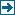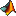Master index Index for .# Index for .

## Matlab files in this directory:Contents sfrmat3 and supporting functions 24 July 2009ahamming [data] = ahamming(n, mid)cent Usage: [b] = cent(a, center)centerfig pos=centerfig(relwidth,relheight,scale) calculates the Position vector to center a figure on the screencentroid function [loc] = centroid(x)clip out= clip(in, low, high)clipping [n, status] = clipping(a)deriv1 [b] = deriv1(a, nlin, npix, fil)findedge [slope, int] = findedge(cent, nlin)findfreq [freqval, sfrval] = findfreq(dat, val, imax, fflag) find frequency for specifiedfir2fix [correct] = fir2fix(n, m);getoecf [array, status] = getoecf(array, oename) applies oecf LUTgetroi [select, coord] = getroi(array, dialtext, tol) Select and return region of interestimageread [status,dat,ftype,fpath,fname] = imageread(fname, nlin, npix) reads image files;inbox1 Dialog box for input of data sampling for SFR calculationinbox3 Dialog box for input of data sampling and weights for red, greenisarray out = isarray(a)project [point, status] = project(bb, loc, slope, fac)readraw1 [dat1] = readraw1(fn, nbyte, nlin, npix, ncol) read images stored as rawreadrawgui [nbyte, nlin, npix, ncol] = readrawgui(filename, nbyte, nlin, npix, ncol)results2 results: Matlab function saves results computed by sfrmat3.m.rotate90 [out] = rotate90(in, n) 90 degree counterclockwise rotations of matrixrotatev [a, nlin, npix, rflag] = rotatev(a)rotatev2 [a, nlin, npix, rflag] = rotatev2(a) Rotate edge array verticalsampeff [eff, freqval, sfrval] = sampeff(dat, val, del, fflag, pflag) Sampling efficiency from SFRsfrmat3 MatLab function: sfrmat3 Slanted-edge Analysis for digital camera and scannersplash info: displays simple splash window announcing program name, versionstd2 std2 standard deviation of matrix elements.test_sfrmat3 Test sfrmat3 and compare with sfrmat2textfig [] = textfig(dat,h) puts text in figure

## Subsequent directories:

• data
• doc

Generated on Mon 27-Jul-2009 13:44:15 by m2html © 2003